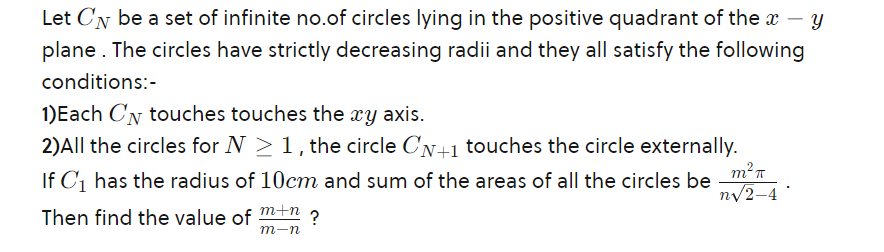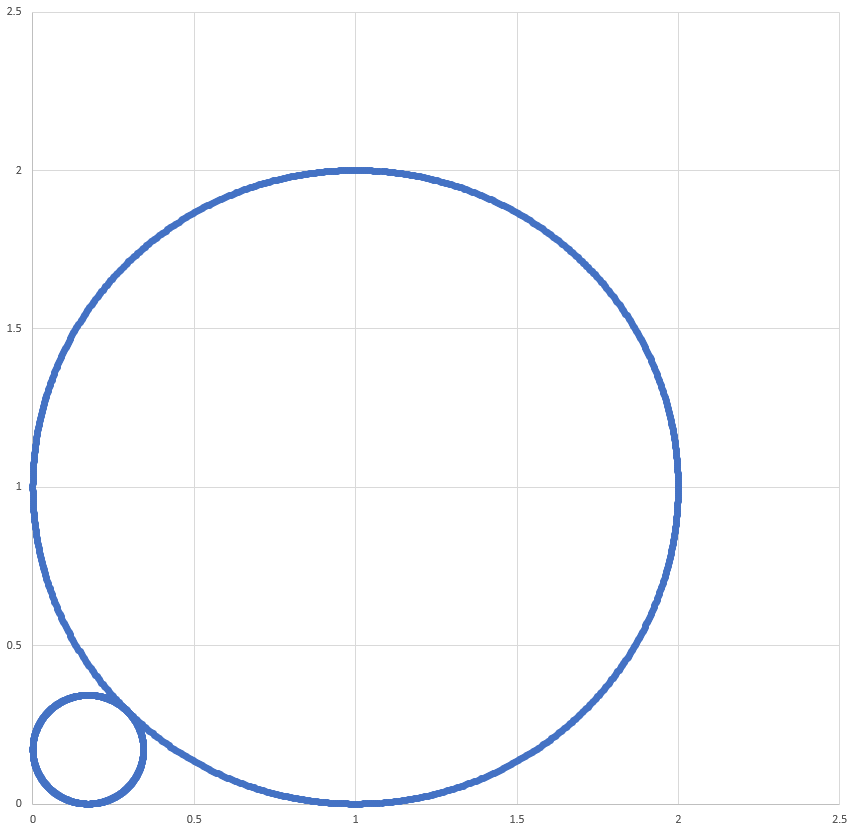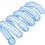# Circle Area Geometric Series

Here is a question I was asked recently (click for zoomed-in version).Let's look at a case where the first circle has a radius of $1$. It is a scaled version of the problem under consideration.The center of the big circle is at $(1,1)$, the center of the little circle is at $(a,a)$, and the point of contact is $\Big(1 - \frac{1}{\sqrt{2}},1 - \frac{1}{\sqrt{2}} \Big)$. We know that the radius of the small circle is equal to the distance from the center of the small circle to the point of contact.

$a^2 = \Big(a - 1 + \frac{1}{\sqrt{2}} \Big)^2 + \Big(a - 1 + \frac{1}{\sqrt{2}} \Big)^2$

Solving for $a$ yields $a = 3 - 2 \sqrt{2}$. The ratio of the area of the small circle to the big circle is $a^2 = 17 - 12 \sqrt{2}$.

Now back to the problem at hand. The area of the circle of radius $10$ is $100 \pi$. The remaining circles follow in an infinite geometric series with common ratio $17 - 12 \sqrt{2}$. The total area is therefore:

$A = \frac{100 \pi}{1 - (17 - 12 \sqrt{2})} = \frac{100 \pi}{12 \sqrt{2} - 16} = \frac{25 \pi}{3 \sqrt{2} - 4}$Note by Steven Chase
1 year, 1 month ago

This discussion board is a place to discuss our Daily Challenges and the math and science related to those challenges. Explanations are more than just a solution — they should explain the steps and thinking strategies that you used to obtain the solution. Comments should further the discussion of math and science.

When posting on Brilliant:

• Use the emojis to react to an explanation, whether you're congratulating a job well done , or just really confused .
• Ask specific questions about the challenge or the steps in somebody's explanation. Well-posed questions can add a lot to the discussion, but posting "I don't understand!" doesn't help anyone.
• Try to contribute something new to the discussion, whether it is an extension, generalization or other idea related to the challenge.

MarkdownAppears as
*italics* or _italics_ italics
**bold** or __bold__ bold
- bulleted- list
• bulleted
• list
1. numbered2. list
1. numbered
2. list
Note: you must add a full line of space before and after lists for them to show up correctly
paragraph 1paragraph 2

paragraph 1

paragraph 2

[example link](https://brilliant.org)example link
> This is a quote
This is a quote
    # I indented these lines
# 4 spaces, and now they show
# up as a code block.

print "hello world"
# I indented these lines
# 4 spaces, and now they show
# up as a code block.

print "hello world"
MathAppears as
Remember to wrap math in $$ ... $$ or $ ... $ to ensure proper formatting.
2 \times 3 $2 \times 3$
2^{34} $2^{34}$
a_{i-1} $a_{i-1}$
\frac{2}{3} $\frac{2}{3}$
\sqrt{2} $\sqrt{2}$
\sum_{i=1}^3 $\sum_{i=1}^3$
\sin \theta $\sin \theta$
\boxed{123} $\boxed{123}$

Sort by:

@Steven Chase Thanks you so much sir.

- 1 year, 1 month ago

@Steven Chase BTW how do you predicted that they will follow geometric progression?

- 1 year, 1 month ago

Because the small circle just becomes the new big circle, and the whole thing repeats again

- 1 year, 1 month ago

Clever deduction. Nice!

- 1 year, 1 month ago